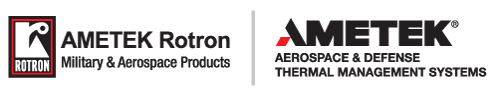Skip to content

# Cooling Flow Calculation

Forced air cooling of electronic components results in a dramatic improvement over natural convection cooling. This effectiveness is the result of the following:
• Reduction in the temperature of the air in the vicinity of the components being cooled.
• Improvement in the heat transfer coefficient of the components transferring heat to the air surrounding them.

Although the best way to make an accurate determination of cooling requirements is by actual test of the equipment to be cooled; a good approximation of the amount of air required can be determined from the mass flow relationship:

q=wCpΔt          (Eq. 1)

 Where q = amount of heat absorbed by the air in BTU/hr w = mass flow of air in lb/hr Cp = specific heat of air in BTU/lb °F Δt = temperature rise of the air in °F

This equation yields the following formula, which is more directly applied to electronics forced air-cooling:

Q = (178.4*ti*kW)/(Δt*Pb)          (Eq. 2)

 Where Q = airflow required in cubic feet per minute ti = inlet temperature in R (R = °F + 460°) Δt = temperature rise across the equipment in °F kW = power to be dissipated in the equipment in kilowatts Pb = barometric pressure at the air inlet in inches of Hg

Here it is assumed that all of the heat to be dissipated is picked up by the air; i.e. conduction and radiation as well as natural convection effects on the external surfaces of the equipment are ignored.

For standard conditions of 70°F and 29.92" Hg, equation 2 reduces to the familiar:

Q = (3160*KW)/ Δt          (Eq. 3)

Recognizing that a given cooling application has numerous design considerations, a temperature rise of 15°F will usually yield effective cooling without incurring penalties of over sizing of the air moving device.

For a general reference on the subject, consult the book Cooling of Electronic Equipment by Allen W. Scott, John Wiley & Sons, New York, NY, 1974.